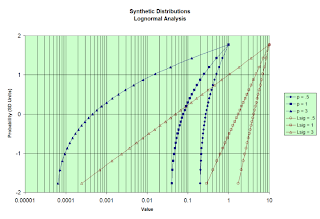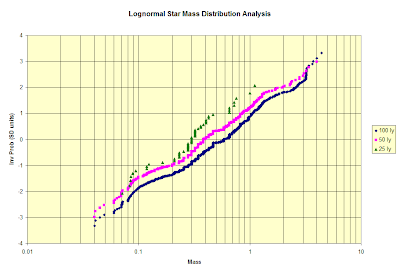## Tuesday, January 06, 2009

### Visual analysis of star mass distribution

kw: analysis, statistical distributions, stars, lognormal, power law

Below I analyze the mass distribution of a couple thousand of the nearest stars. I must first explain what is going on here. It has to do with finding a way to chart a series of data so that they approximate a straight line. This is called linearizing the geodesic.

One of my nerdy obsessions is gathering examples that illustrate the Theory of Breakage. The premise was proven mathematically by Kolmogoroff in 1941 (published in German) and offered to the English-reading public in The Lognormal Distribution by J. Aitchison and J.A.C. Brown in 1957. If you drop a brittle object onto a hard surface so it shatters, then weigh every piece, an analysis would show that the weights of the pieces are distributed lognormally. The analysis of clastic sediments follows lognormal reasoning.

Rather than foist a mathematical proof on you here, I'll support the idea conceptually. The lognormal distribution is based on the normal, or Gaussian, distribution, the famous "bell curve." The relation is thus: If you take the logarithm of a set of items which are distributed lognormally, the resulting distribution will be normal. These six numbers make up a very small normal sequence: 7, 8.4, 9.5, 10.5, 11.6, 13. The mean (and median) value for this distribution is 10. If you add the first and last numbers you get 20, twice the median; so if you add any pair of numbers equally distant from the "gap" between the 9.5 and the 10.5, you get twice the median.

If we take an exponential function of these numbers, we get a lognormal distribution. In this case, let us take 2 to the power of each of the numbers, and divide the resulting series by 100. Then these six numbers form a very small lognormal sequence: 1.29, 3.4, 7.2, 14.5, 30.7, 81.3. As these are lognormally distributed (for they were constructed so), the median is a logmedian, formed by multiplying the extrema and taking the square root: SQRT(1.29*81.3) = 10.2. Again, this works with any pair of numbers equidistant from the "gap" between the 7.2 and the 14.5.

Where a normal distribution is characterized by values clustered about a median value, a lognormal distribution has a cluster on the small side of the median, and a scattering of the largest values, in other words, a heavy tail. In many cases, the largest member of a lognormal sequence is larger than the sum of the rest of the terms.

A bunch of random items that are related by a similar additive process tend to be normally distributed. The Central Limit Theorem, upon which Kolmogoroff's proof is based, states that repeatedly taking the sum of several random numbers produces a normal distribution. Exponentiation turns addition into multiplication, so by the same reasoning, repeatedly taking the product (or the quotient) of several random numbers produces a lognormal distribution. Thus, in nature, additive (and subtractive) processes produce normally distributed values, while multiplicative or divisive processes (exemplified by breakage) produce lognormally distributed values.

I got interested in this subject when I read (I don't recall where) of an alternative hypothesis of breakage, that the resulting pieces might constitute a "scale free" or "power law" distribution. A power law distribution somewhat resembles a lognormal distribution, in that there are many small members and few large ones. However, the quantity of small members is quite a bit greater. A power law sequence is most simply produced by dividing a series of numbers into a constant, but all practical sequences are produced by dividing some power of each member of the series into a constant.

A very small power law sequence, produced by dividing 30 by 6, 5, 4, 3, 2 & 1, is
5, 6, 7.5, 10, 15, 30. Using the generating law to determine the median, we find it is 30/3.5 = 8.57 (The procedure used for a lognormal sequence yields 8.66). The drawback to continuing this series is that it has no finite sum. A power law sequence from a distribution that will converge to a finite sum is found by dividing the 3/2 power of the first six numbers into 30: 2.04, 2.7, 3.8, 5.8, 10.6, 30. This has median 4.6.

While there are software routines that can distinguish whether a sequence is distributed according to a power law or lognormally (and a great many others), there is a simple visual test that I like to administer. The illustrations that follow have larger-sized versions "behind" them, available by clicking. I prepared these using Microsoft Excel, which unfortunately doesn't have a probability axis option for its charting tools, so I use a transformation to linearize a probability axis.I illustrate the technique here; I generated six sample distributions of 25 members each. Three are power law sequences and three are lognormal sequences. The latter were scaled to have a largest member equal to 10, so we can see their shapes in this chart and the one that follows.

This chart presentation is log-log. On such a chart a power law sequence plots as a straight line. The three blue lines are power law sequences, and the three dark red lines are lognormal sequences with different breadths of distribution (different values of the logvariance). The strong curvature of the red lines indicates that they are far from linearized in this type of chart.This chart takes a standard normal sequence as the vertical axis; the value "1" means one Standard Deviation from the mean (1σ or "one sigma"). 25 values have probabilities ranging from 0.04 to 0.96, which are from -1.75σ to +1.75σ. The horizontal axis plots the values of the members of the six distributions, and is shown in logarithmic transformation. As before, the blue lines are the power law sequences and the red lines are the lognormal sequences.

Now it is the latter that plot in a straight line on these axes, while the power law sequences plot as distinct curves. This is diagnostic for each type of data; they plot as a straight line on appropriate axes, and as a curve under any other transformation.Now we can plot the stars' masses. I gathered stellar data from many sources, but I'd like to acknowledge the Nearby Star Observers for their work and for a great collection of links that allowed me to find some of the sources of data I used.

I gathered information on mass where I could, but for most of the stars I used databases that had the stars' spectral and luminosity types, distances and magnitudes, and applied stellar evolution theory to approximate the masses. I eventually had a list of the masses of 2,200 stars out to a distance of 100 light-years. I plotted these, the 688 stars closer than 50 light years, and the 51 stars closer than 25 light years, in log-log coordinates for this chart. Of the 2,200 stars, 1,876 are on the Main Sequence (Class V), and are distributed spectrally as follows:
• B - 2
• A - 38
• F - 50
• G - 379
• K - 578
• M - 829
These accord well with other analyses of the relative number of each type of star. The total list also includes some giants and close to 100 white dwarfs, plus a very few brown dwarfs. For the white dwarfs I did my best to infer the mass of the star when it was younger, because the aim here is to investigate the breakup of the gas-and-dust clouds that formed these stars.

The clear conclusion from this plot is that the data I have are not distributed according to a power law. There are two possible reasons: a great many K- and M-type stars may yet be discovered within 100 light years of Earth, or the actual distribution is not power law.A lognormal analysis yields much straighter lines. Let us focus on the pinkish line. This set of 688 stars is probably very nearly complete.

A sphere twice the radius of another ought to have eight times as many stars within it, compared to the other. But 2,200/688 = 3.2, so many stars in the 50-100 light-year range are probably not discovered yet, and most of them will be small, dim stars (K and M) and brown dwarfs. On the other hand, 688/51 = 13.5, so there is a dearth of stars in the solar neighborhood. This is known from the literature about the "solar bubble".

What does this chart show us? It indicates to me that the distribution of star mass is most likely lognormal, and if so we can infer a few things. Projecting a straight line through the pink sequence to the 4σ lines indicates that in a complete sample of about 32,000 stars there ought to be a smattering of giants with up to 9 or 10 solar masses, and similar smattering of M9 types and brown dwarfs as small as 0.02 solar masses, about 20 Jupiter masses. Extrapolating wildly to 7σ (almost a trillion stars, close to the probable number in the Galaxy), puts us in a realm in which the heaviest star approaches 100 solar masses and the lightest brown dwarfs are no more than twice the mass of Jupiter. These conclusions seem plausible, so I have considerable confidence in a lognormal distribution of stellar mass.

#### 1 comment:

Anonymous said...

Interesting. My question is: Why? Is this distribution of stellar masses the result of a. the rate at which stars of different masses are created, b. the rate at which they die, c. the time they exist, d. any 2, or e all 3? My guess is it is purely related to the lifetime of the stars (the most massive having the shortest lifetime and thus being included in an instantaneous count the least), but being less than an ameture, I will leave the proof to the reader. *lol* blagos@tycoint.com